Courses

# Ideal Bose & Fermi Gases (Part-1) - Thermodynamic & Statistical Physics, CSIR-NET Physical Sciences Physics Notes | EduRev

## Physics : Ideal Bose & Fermi Gases (Part-1) - Thermodynamic & Statistical Physics, CSIR-NET Physical Sciences Physics Notes | EduRev

The document Ideal Bose & Fermi Gases (Part-1) - Thermodynamic & Statistical Physics, CSIR-NET Physical Sciences Physics Notes | EduRev is a part of the Physics Course Physics for IIT JAM, UGC - NET, CSIR NET.
All you need of Physics at this link: Physics

Gases
Here we consider systems with the kinetic energy exceeding the potential energy of inter-particle interactions: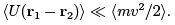Ideal Gases

We start from neglecting the potential energy of interaction completely. Note though that quantum effect does not allow one to consider particles completely independent. The absence of any interaction allows one to treat any molecule as subsystem and apply to it the Gibbs canonical distribution: the average number of molecules in a given state is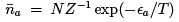which is called Boltzmann distribution. One can also use grand canonical ensemble considering all molecules in the same state as a subsystem with a non-fixed number of particles. Using the distribution (35) with N = na and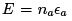one expresses the probability of occupation numbers via the chemical potential: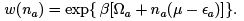Consider now a dilute gas, when all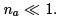. Then the probability of no particles in the given state is close to unity,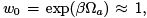and the probability of having one particle and the average number of particles are given by the Boltzmann distribution in the form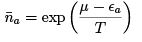(39)

Boltzmann (classical) gas is such that one can also neglect quantum exchange interaction of particles (atoms or molecules) in the same state which requires the occupation numbers of any quantum state to be small, which in turn requires the number of states V p3/h3 to be much larger than the number of molecules N . Since the typical momentum is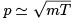we get the condition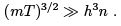(40)

To get the feeling of the order of magnitudes, one can make an estimate with m = 1.6. 10-24g (proton) and n = 1021cm-3 which gives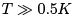Another way to interpret (40) is to say that the mean distance between molecules n-1/3 must be much larger than the wavelength h/p. In this case, one can pass from the distribution over the quantum states to the distribution in the phase space: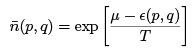(41)
In particular, the distribution over momenta is always quasi-classical for the Boltzmann gas. Indeed, the distance between energy levels is determined by the size of the box,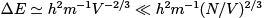which is much less than temperature according to (40). To put it simply, if the thermal quantum wavelength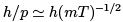is less than the distance between particles it is also less than the size of the box. We conclude that the Boltzmann gas has the Maxwell distribution over momenta. If such is the case even in the external field then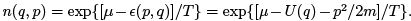That gives, in particular, the particle density in space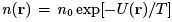where n0 is the concentration without field. In the uniform gravity field we get the barometric formula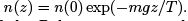Partition function of the Boltzmann gas can be obtained from the partition function of a single particle (like we did for two-level system and oscillator) with the only difference that particles are now real and indistinguishable so that we must divide the sum by the number of transmutations: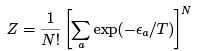Using the Stirling formula ln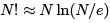we write the free energy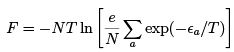(42)
Since the motion of the particle as a whole is always quasi-classical for the Boltzmann gas, one can single out the kinetic energy: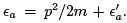If in addition there is no external field (so that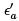describes rotation and the internal degrees of freedom of the particle) then one can integrate over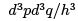and get for the ideal gas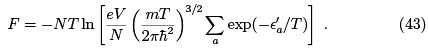To complete the computation we need to specify the internal structure of the particle. Note though that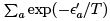depends only on temperature so that we can already get the equation of state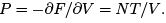Mono-atomic gas. At the temperatures much less than the distance to the first excited state all the atoms will be in the ground state (we put ε0 = 0).
That means that the energies are much less than Rydberg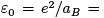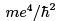and the temperatures are less than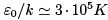(otherwise atoms are ionized).
If there is neither orbital angular momentum nor spin (L = S = 0 — such are the atoms of noble gases) we get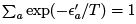as the ground state is non-degenerate and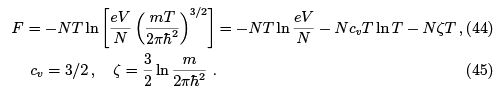Here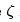is called the chemical constant. Note that for F = AT + B T ln T the energy is linear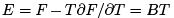that is the specific heat, Cv = B , is independent of temperature. The formulas thus derived allow one to derive the conditions for the Boltzmann statistics to be applicable which requires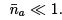. Evidently, it is enough to require exp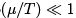where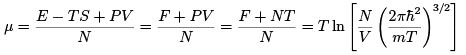Using such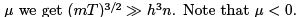If there is a nonzero spin the level has a degeneracy 2S + 1 which adds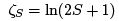to the chemical constant (45). If both L and S are nonzero then the total angular momentum J determines the fine structure of levels εJ (generally comparable with the room temperature — typically,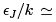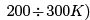Every such level has a degeneracy 2J + 1 so that the respective partition function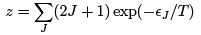Without actually specifying εJ we can determine this sum in two limits of large and small temperature.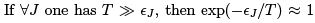and z = (2S + 1)(2L + 1) which is the total number of components of the fine level structure. In this case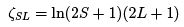In the opposite limit of temperature smaller than all the fine structure level differences, only the ground state with εJ = 0 contributes and one gets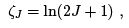where J is the total angular momentum in the ground state.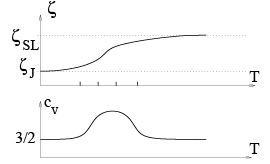Note that cv = 3/2 in both limits that is the specific heat is constant at low and high temperatures (no contribution of electron degrees of freedom) having some maximum in between (due to contributions of the electrons).
We have already seen this in considering two-level system and the lesson is general: if one has a finite number of levels then they do not contribute to the specific heat both at low and high temperatures.

Specific heat of diatomic molecules. We need to calculate the sum over the internal degrees of freedom in (43). We assume the temperature to be smaller than the energy of dissociation (which is typically of the order of electronic excited states). Since most molecules have S = L = 0 in the ground state we disregard electronic states in what follows. The internal excitations of the molecule are thus vibrations and rotations with the energy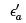characterized by two quantum numbers, j and K :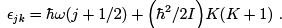(46)

We estimate the parameters here assuming the typical scale to be Bohr radius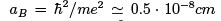and the typical energy to be Rydberg ε=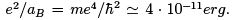Note that m = 9 . 10-28g is the electron mass here. Now the frequency of the atomic oscillations is given by the ratio of the Coulomb restoring force and the mass of the ion: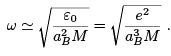Rotational energy is determined by the moment of inertia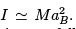We may thus estimate the typical energies of vibrations and rotations as follows: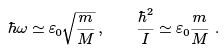(47)

Since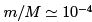then that both energies are much smaller than the energy of dissociation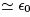and the rotational energy is smaller than the vibrational one so that rotations start to contribute at lower temperatures: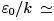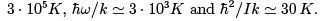The harmonic oscillator was considered in in Sect. 1.4.2. In the quasiclassical limit,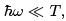the partition function of N independent oscillators is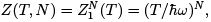the free energy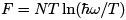and the mean energy from (24): E = N T . The specific heat CV = N .

For a quantum case, the energy levels are given by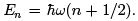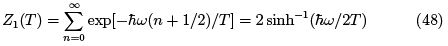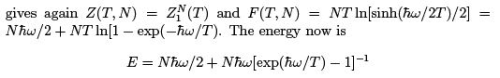where one sees the contribution of zero quantum oscillations and the breakdown of classical equipartition. The specific heat (per molecule) of vibrations is thus as follows: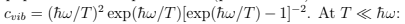we have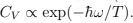At large T we have classical equipartition (every oscillator has two degrees of freedom so it has T in energy and 1 in CV ).
To calculate the contribution of rotations one ought to calculate the partition function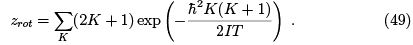Again, when temperature is much smaller than the distance to the first level,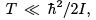the specific heat must be exponentially small. Indeed, retaining only two first terms in the sum (49), we get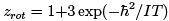which gives in the same approximation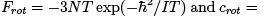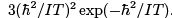We thus see that at low temperatures diatomic gas behaves an mono-atomic.

At large temperatures,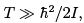the terms with large K give the main contribution to the sum (49). They can be treated quasi-classically replacing the sum by the integral: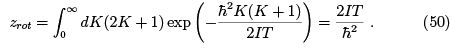That gives the constant specific heat crot = 1. The resulting specific heat of the diatomic molecule,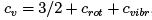is shown on the figure: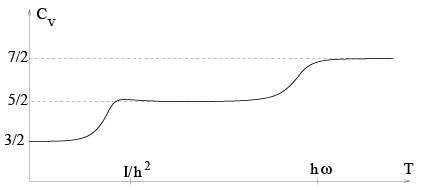Note that for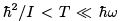the specific heat (weakly) decreases because the distance between rotational levels increases so that the level density (which is actually cv ) decreases.
For (non-linear) molecules with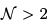atoms we have 3 translations, 3 rotations and 6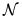— 6 vibrational degrees of freedom (3n momenta and out of total 3n coordinates one subtracts 3 for the motion as a whole and 3 for rotations). That makes for the high-temperature specific heat cv = ctr + crot + cvib = 3=2 + 3=2 + 3- 3 = 3. Indeed, every variable (i.e. every degree of freedom) that enters ε(p, q), which is quadratic in p, q, contributes 1/2 to cv . Translation and rotation each contributes only momentum and thus gives 1/2 while each vibration contributes both momentum and coordinate (i.e. kinetic and potential energy) and gives 1.

159 docs

,

,

,

,

,

,

,

,

,

,

,

,

,

,

,

,

,

,

,

,

,

,

,

,

;